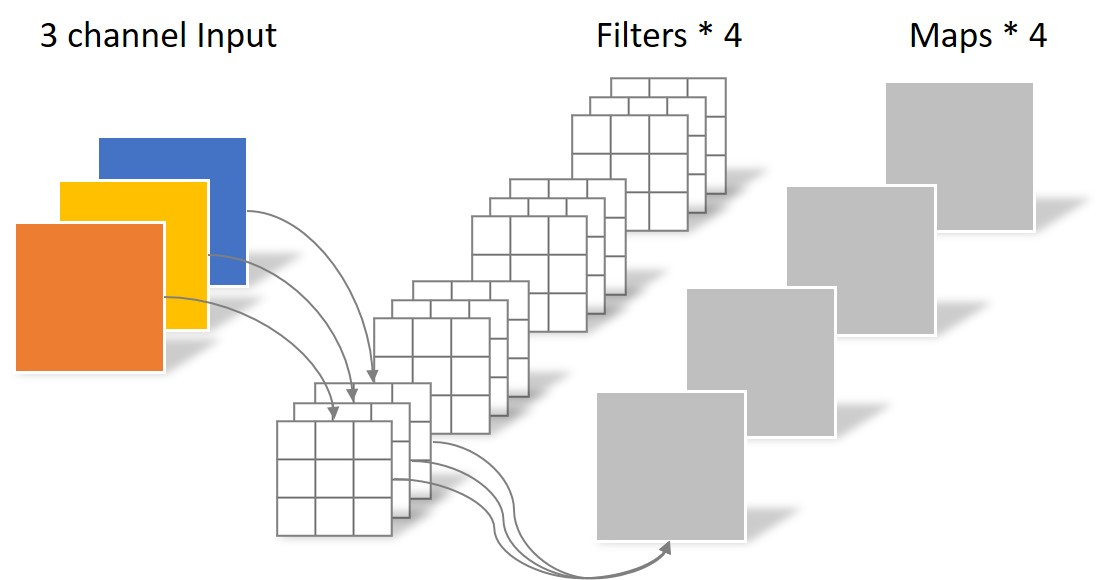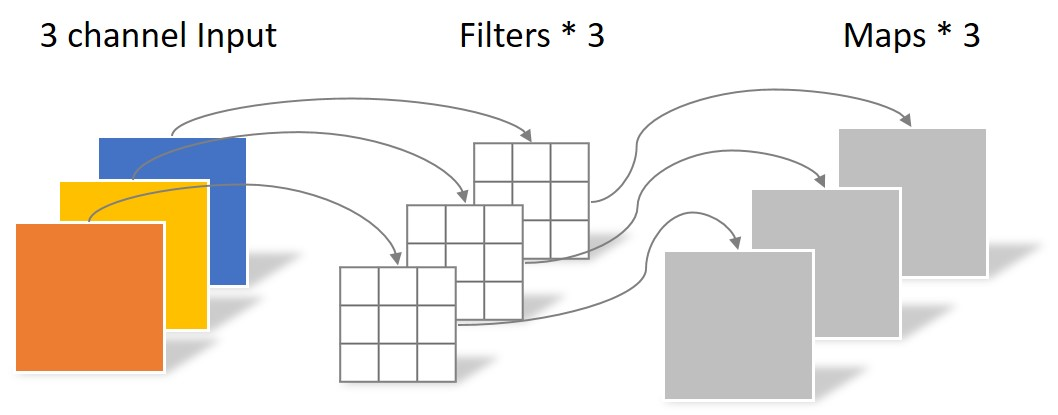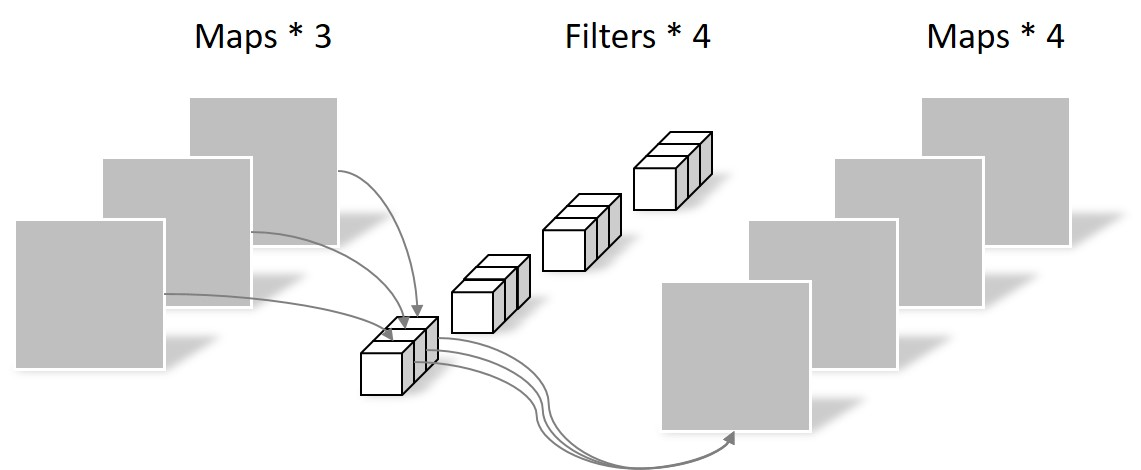# 卷积神经网络中的Separable Convolution

## 常规卷积运算``````N_std = 4 × 3 × 3 × 3 = 108
``````

## Separable Convolution

### Depthwise Convolution``````N_depthwise = 3 × 3 × 3 = 27
``````

Depthwise Convolution完成后的Feature map数量与输入层的depth相同，但是这种运算对输入层的每个channel独立进行卷积运算后就结束了，没有有效的利用不同map在相同空间位置上的信息。因此需要增加另外一步操作来将这些map进行组合生成新的Feature map，即接下来的Pointwise Convolution。

### Pointwise Convolution

Pointwise Convolution的运算与常规卷积运算非常相似，不同之处在于卷积核的尺寸为 1×1×M，M为上一层的depth。所以这里的卷积运算会将上一步的map在深度方向上进行加权组合，生成新的Feature map。有几个Filter就有几个Feature map。如下图所示。``````N_pointwise = 1 × 1 × 3 × 4 = 12
``````

## 参数对比

``````N_std = 4 × 3 × 3 × 3 = 108
``````

Separable Convolution的参数由两部分相加得到：

``````N_depthwise = 3 × 3 × 3 = 27
N_pointwise = 1 × 1 × 3 × 4 = 12
N_separable = N_depthwise + N_pointwise = 39
``````